# ASVAB Math Study Guide Part 2

## Fractions, Percentages, and Decimals

This is the second part of our free ASVAB Math Study Guide. This part covers fractions, percentages and decimals. Read through the material carefully and be sure to study the examples that are given. At the bottom of the page there is a review quiz to test your knowledge of these topics.

### Multiplying Fractions

Recall that the top number in a fraction is called the numerator and the bottom number is called the denominator:

$\dfrac{\text{Numerator}}{\text{Denominator}}$

Suppose we want to multiply the following fractions:

$\dfrac{2}{3} × \dfrac{4}{9}$

Multiply the numerators to find the numerator of the answer. Multiply the denominators to find the denominator of the answer:

$\dfrac{2}{3} × \dfrac{4}{9}$ $= \dfrac{2 × 4}{3 × 9}$ $= \dfrac{8}{27}$

Always reduce the fraction to lowest terms if possible. In this case, because the numerator and denominator share no factors in common (e.g. we cannot divide both by 2) the fraction cannot be reduced.

### Dividing Fractions

Suppose we want to divide the following fractions:

$\dfrac{1}{3} ÷ \dfrac{4}{5}$

Begin by converting the division problem into a multiplication problem.

We do this by changing the divide (÷) into a multiply (×) and flipping the second fraction over (taking its reciprocal).  When you see fractions being divided think “Flip It and Multiply”:

$\dfrac{1}{3} ÷ \dfrac{4}{5} \;$ becomes $\; \dfrac{1}{3} × \dfrac{5}{4}$

Using the Multiplication technique from above:

$\dfrac{1}{3} × \dfrac{5}{4}$ $= \dfrac{1×5}{3×4}$ $= \dfrac{5}{12}$

This fraction can be reduced no further.

### Adding and Subtracting Fractions with a Common Denominator

When adding or subtracting fractions, the fractions must first be changed so that they share a common denominator—the same number in the denominator—if they do not already.

In this first example the denominator is already the same in both fractions; in other words they share a common denominator.

$\dfrac{2}{5}+\dfrac{1}{5}$

When adding fractions with a common denominator, we add the numerators, but leave the denominators the same:

$\dfrac{2}{5} + \dfrac{1}{5}$ $= \dfrac{2 + 1}{5}$ $= \dfrac{3}{5}$

Subtracting fractions with a common denominator follows a similar approach.  We subtract the numerators, but leave the denominators the same:

$\dfrac{2}{5} − \dfrac{1}{5}$ $= \dfrac{2 − 1}{5}$ $= \dfrac{1}{5}$

### Adding and Subtracting Fractions with Different Denominators

Let’s now consider an example where the denominators of the fractions being added are not the same:

$\dfrac{2}{5}+\dfrac{1}{3}$

The first step is to find a common denominator, a number that is a multiple of both of the denominators.

15 is a common multiple of both 5 and 3, so it would be a good choice for our common denominator. We could also have used 30, but the lower the common multiple is, the less reducing we will need to perform at the end.

We have found our common denominator, but now we need to convert each of the original fractions to use the common denominator.

We have two fractions. Let’s start with $\dfrac{2}{5}$.

What do we need to multiply the denominator by to change it to our common denominator of 15?

$5~×~? = 15$

We need to multiply it by 3. But whatever we do to the denominator, we must also do to the numerator:

$\dfrac{2}{5}$ $= \dfrac{2×3}{5×3}$ $= \dfrac{6}{15}$

We need to also convert our second fraction using the same approach:

$\dfrac{1}{3}$ $= \dfrac{1×5}{3×5}$ $= \dfrac{5}{15}$

We can now rewrite the original problem into one with fractions sharing a common denominator:

$\dfrac{2}{5}+\dfrac{1}{3}$ $= \dfrac{6}{15}+\dfrac{5}{15}$ $= \dfrac{6+5}{15}$ $= \dfrac{11}{15}$

Subtracting follows a similar process:

$\dfrac{2}{5}−\dfrac{1}{3}$ $= \dfrac{6}{15}−\dfrac{5}{15}$ $= \dfrac{6−5}{15}$ $= \dfrac{1}{15}$

### Mixed Fractions (aka “Mixed Numbers”)

Consider the following mixed number:

$3\dfrac{2}{5}$

It means “$3$ wholes + $\dfrac{2}{5}$“

To work with mixed numbers, it is typically best to convert them to an improper fraction (a special type of fraction where the numerator is greater than the denominator).

To convert $3\frac{2}{5}$ to an improper fraction, we must first determine what the new numerator will be. We do so by multiplying the whole number by the denominator and add the numerator to it:

$5 × 3 + 2 = 15 + 2 = 17$

The denominator of the improper fraction will be the same as the denominator from the original mixed number $= 5$.

$\dfrac{17}{5}$

### Converting Percentages to Decimals (and vice versa)

The percentage symbol, %, literally means “per hundred (100)”.

So, 12% means “12 per 100”.  We can write “12 per 100” as the fraction $\frac{12}{100}$.

Taking this a step farther, we can convert 12% into a decimal by simply moving the decimal point two positions to the left: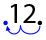$12\% = 0.12$

Let’s try one more. Convert 7.5% to a decimal: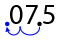Notice that we must fill any “gaps” with the 0 digit.

$7.5\% = 0.075$

Now let’s look at converting from a decimal to a percentage.

Convert 0.371 to a percentage: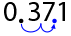$0.371 = 37.1\%$

Convert 0.3 to a percentage: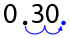Again, we moved the decimal two positions to the right, but this time we needed to fill the “gaps” with the 0 digit.

$0.3 = 30\%$

### Percentage Calculations

Let’s say we have been asked to find 30% of 120.

When you see “of”, translate it as “times”.

$30\% \text{ of } 120 = 30\% × 120$

Next, we must convert the percentage to a decimal.

$30\% = 0.3$

$30\% × 120 = 0.3 × 120$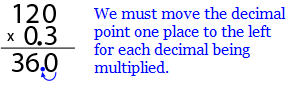Suppose we want to calculate the sales tax on an item that costs $49. Assume our sales tax is 7.5%. How do we find 7.5% of \$49?

$7.5\% \text{ of } \$49 = 7.5\% × \$49 = 0.075 × \$49$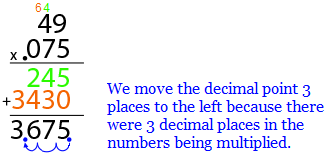$7.5\% \text{ of } \$49 = \$3.675$(Which would be rounded to$\$3.68$)

### Converting Improper Fractions to Mixed Numbers

Though Improper Fractions are generally easier to work with, there are times when you will be interested in converting them to a Mixed Number.

Consider the following Improper Fraction:

$\dfrac{23}{5}$

To convert this to a Mixed Number, we divide the numerator by the denominator:

$23 ÷ 5 = 4$ with a remainder of $3 = 4~r3$

In other words, $5$ goes into $23$ four times with $3$ left over.

We take the $4$ and put it as the whole number, the remainder of $3$ becomes the numerator of the fraction, and the denominator of the fraction remains unchanged as $5$:

$4 \dfrac{3}{5}$

Now that you’ve read more of our lessons and tips for the Math section of the ASVAB, put your skills to practice with the review quiz below. Try not to reference the above information and treat the questions like a real test.

## Part 2 Review Quiz:

Congratulations - you have completed .

You scored %%SCORE%% out of %%TOTAL%%.

Your performance has been rated as %%RATING%%

 Question 1

### Convert $.068$ to a percentage.

 A $68\%$ B $6.8\%$ C $.68\%$ D $0.068\%$
Question 1 Explanation:
To convert a decimal to a percentage, move the decimal point two numbers to the right. $.068$ becomes $6.8\%$.
 Question 2

### $\dfrac{5}{6} ÷ \dfrac{2}{3} =$ Hint: remember to convert any improper fractions to mixed numbers

 A $1 \dfrac{1}{4}$ B $1 \dfrac{3}{12}$ C $\dfrac{5}{4}$ D $\dfrac{15}{12}$
Question 2 Explanation:
To solve a division problem, you need to flip the second fraction and then multiply. The new problem will look like this:

$\dfrac{5}{6} × \dfrac{3}{2}$

Next, multiply the numerators and the denominators. $5 × 3 = 15$ and $6 × 2 = 12$, so the resulting fraction is $\frac{15}{12}$. Since both numbers are divisible by $3$, the fraction can be simplified to $\frac{5}{4}$.

It is still an improper fraction. To convert it to a mixed number, we evaluate how many times $4$ can go into $5$ (once) and make that our whole number. Since that leaves an extra fourth, our final mixed number is $1 \dfrac{1}{4}$.
 Question 3

### $\dfrac{8}{10} + \dfrac{4}{10} − \dfrac{2}{10} =$ Hint: remember to simplify your answer to the lowest possible terms

 A $1$ B $\dfrac{6}{10}$ C $\dfrac{10}{10}$ D $10$
Question 3 Explanation:
Since the denominator is the same on all the fractions, you only have to add and subtract the numerators. $8 + 4 = 12$, and subtracting $2$ gets us $10$. Since $\frac{10}{10}$ can be simplified to $1$, which is the correct answer.
 Question 4

### $\dfrac{7}{8} − \dfrac{1}{3} =$ Hint: remember to simplify your answer to the lowest possible terms

 A $\dfrac{4}{8}$ B $\dfrac{13}{24}$ C $\dfrac{26}{48}$ D $\dfrac{6}{5}$
Question 4 Explanation:
To solve a subtraction problem with different denominators, we need to change one or both of the fractions so the denominators are the same.

Since the lowest common multiple of $8$ and $3$ is $24$, we can multiply the numerator and denominator of $\frac{7}{8}$ by $3$ to get $\frac{21}{24}$.

Then we can multiply the numerator and denominator of $\frac{1}{3}$ by $8$ to get $\frac{8}{24}$.

Our new subtraction problem looks like this:

$\dfrac{21}{24} − \dfrac{8}{24}$.

Now, all we have to do is subtract the numerators:

$21 − 8 = 13$

$\dfrac{13}{24}$ can't be simplified, so that's our final answer.
 Question 5

### Convert $4 \dfrac{2}{3}$ into an improper fraction.

 A $\dfrac{14}{3}$ B $\dfrac{4}{3}$ C $\dfrac{11}{3}$ D $\dfrac{8}{3}$
Question 5 Explanation:
You can find an improper fraction's numerator by multiplying the whole number by the denominator, and then adding the numerator:

$4 × 3 + 2 = 14$

Since the denominator stays the same, the improper fraction is simply $\frac{14}{3}$.
 Question 6

### Add $0.31 + 0.47$.

 A $78$ B $.78\%$ C $7.8\%$ D $78\%$
Question 6 Explanation:
Remember that when a decimal becomes a percentage, the decimal point moves over two spots to the right. Thus, $.78$ becomes $78\%$.
 Question 7

### $\dfrac{7}{8} × \dfrac{4}{5} =$

 A $\dfrac{14}{20}$ B $\dfrac{28}{45}$ C $\dfrac{7}{10}$ D $\dfrac{12}{7}$
Question 7 Explanation:
When multiplying fractions, multiply the numerators together and the denominators together. Since $7 × 4 = 28$ and $8 × 5 = 40$, the resulting fraction is $\frac{28}{40}$.

This, however, is not simplified. $28$ and $40$ are both divisible by $2$ and $4$. Since we want the fraction to be reduced as much as possible, we will divide each number by $4$ and get the correct answer of $\frac{7}{10}$.
 Question 8

### $1 \dfrac{1}{3} × 4 \dfrac{5}{6} =$ Hint: remember to simplify your answer to the lowest possible terms

 A $\dfrac{116}{18}$ B $\dfrac{232}{6}$ C $6 \dfrac{4}{9}$ D $4 \dfrac{5}{18}$
Question 8 Explanation:
To solve this problem, we can start by turning the mixed numbers into improper fractions. Multiply the whole number by the denominator and add the numerator to find the new numerator. The first fraction can be solved with the following equation:

$1 × 3 + 1 = 4$

Keep the original denominator. Thus, your new fraction is $\frac{4}{3}$.

The second improper fraction can be found by the following:

$4 × 6 + 5 = 29$

Keep the original denominator. Thus, your new fraction is $\frac{29}{6}$.

Now, multiply the two new fractions like normal, numerator by numerator and denominator by denominator.

$\dfrac{4}{3} × \dfrac{29}{6} = \dfrac{116}{18}$

We can simplify the improper fraction and then convert it back into a mixed number. The greatest common factor of $116$ and $18$ is $2$, so dividing the numerator and denominator by $2$ gives us $\frac{58}{9}$.

To convert the answer back into a mixed number, find how many times $9$ can go into $58$. The answer, $6$, becomes our whole number. The remainder, $4$, becomes our new numerator, and the denominator stays the same. This gives us $6 \frac{4}{9}$.
 Question 9

### $32\%$ of $106$ is

 A $33.92$ B $3,392$ C $138$ D $13.8$
Question 9 Explanation:
To find the percentage of a number, first turn the percent into a decimal. $32\%$ becomes $0.32$. Then, multiply to get the answer.

$0.32 × 106 = 33.92$
 Question 10

### A shirt costs $\$22$. If there's a$5.5\%$sales tax, how much will you pay for the shirt?  A$\$1.21$ B $\$12.1$C$\$23.21$ D $\$27.50$Question 10 Explanation: First, convert the percentage into a decimal by moving the decimal point two spots to the left to get$0.055$. Multiply the decimal by the original price:$22 × .055 = 1.21$This is not our final answer, however.$1.21$is the amount we have to add to to original price. Thus, the final answer is found with:$22 + 1.21 = 23.21\$
Once you are finished, click the button below. Any items you have not completed will be marked incorrect.
There are 10 questions to complete.
 ← List →